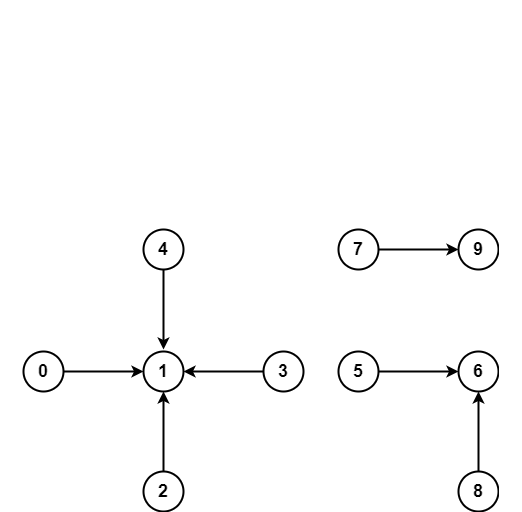## Remarks

• Union Find is a data structure keeps track of a set of elements that are partitioned into a number of disjoint subsets.
• It has two operations union(p, q) and find(p).
• the find/search finds the subset element p belongs to.
• and union/merge merges the subsets containing p and q.
• It memory usage is O(N), and each find and union operations are near O(1) in time.
• The algorithm can be used to find all connected components in a network. Compare to DFS/BFS for finding connected components, UF is better suited for dynamic connectivity where edges can be added overtime and the components info can be queried at any given time.
• It is also used in kruskal’s algorithm to find the minimal spanning tree for a graph.

### Implementation

Simplest implementation to use the core of union find.

parents = list(range(n))

def find(i):
if i != parents[i]:
parents[i] = find(parents[i])
i = parents[i]
return parents[i]

def union(x, y):
x, y = find(x), find(y)
if x == y: return
parents[x] = y
return y


Define a class for UF

class UnionFind:
def __init__(self, n):
self.parents = list(range(n))
self.sizes =  * n

def _find_recursive(self, i):
while i != self.parents[i]:
# path compression, have i points to the cluster centroid
self.parents[i] = self._find_recursive(self.parents[i])
i = self.parents[i]
return i

def _find_iterative(self, i):
# 1 path to find the root
root = i
while self.parents[root] != root:
root = self.parents[root]
# 2 path to assign every node in the path to points at root
while self.parents[i] != root:
parent = self.parents[i]
self.parents[i] = root
i = parent
return root

find = _find_recursive

def union(self, p, q):
"""Attempt to union two elements

Args:
p, q: two elements to be attempted for union

Returns:
Boolean indicates whether a union operation is performed.
"""
root_p, root_q = map(self.find, (p, q))
if root_p == root_q: return False
small, big = sorted([root_p, root_q], key=lambda x: self.sizes[x])
self.parents[small] = big
self.sizes[big] += self.sizes[small]
return True


Use Union Find to find connected components in undirected graph.

connections = [[0, 1], [1, 2], [2, 3], [3, 4], [5, 6], [6, 8], [7, 9]]

uf = UnionFind(10)
for p, q in connections: uf.union(p, q)
num_components = len(set(uf.find(i) for i in range(10)))
print(num_components)• To find minimum spanning tree in the graph where there are weights on the edges.
1. Sort edges by edge weights ascendingly.
2. Iteratve over the edges unify nodes when two nodes don’t belong to same cluster.
3. Repeat 2 until no nodes or no edges.

### An Implementation without pre-allocation

class UnionFind:
def __init__(self):
self.parents = dict()
self.sizes = dict()
self.n_sets = 0

def __contains__(self, i):
return i in self.parents

def insert(self, i):
if self.__contains__(i): return
self.parents[i] = i
self.sizes[i] = 1
self.n_sets += 1

def find(self, i):
while i != self.parents[i]:
self.parents[i] = self.find(self.parents[i])
i = self.parents[i]
return i

def union(self, p, q):
"""Attempt to union two elements

Returns:
Boolean indicates whether a union operation is performed.
"""
root_p, root_q = map(self.find, (p, q))
if root_p == root_q: return False
small, big = sorted([root_p, root_q], key=lambda x: self.sizes[x])
self.parents[small] = big
self.sizes[big] += self.sizes[small]
self.n_sets -= 1
return True